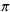Create a new printableGeometry
Math Worksheets

Sample - Click above to make a new math worksheet (PDF).
 Name _____________________________Date ___________________
Perimeter and Area
Find the circumference of each circle. State your answer in terms ofand also round your answer to the nearest tenth.

1.
diameter =
 43
m

2.

3.

4.
 diameter = 12.46 cm

5.

6.

7.
 diameter = 26.38 m

8.
 diameter = 44 m

9.
diameter =
 27
mm

10.
diameter =
 83
m

11.

12.
 diameter = 19.16 mm

13.
 diameter = 34 mm

14.

15.

16.

17.
diameter =
 211
mm

18.
 diameter = 39.06 cm

19.

20.

21.
 diameter = 33.24 m

 22 * This is a pre-made sheet.Use the link at the top of the page for a printable page.
23.
 13
cm

 24 * This is a pre-made sheet.Use the link at the top of the page for a printable page.
25.
 53
mm

26.
 diameter = 29.74 cm

27.
 diameter = 44.24 cm